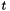## Apophenia

Some examples

Here are a few pieces of sample code for testing your installation or to give you a sense of what code with Apophenia's tools looks like.

Two data streams

The sample program here is intended to show how one would integrate Apophenia into an existing program. For example, say that you are running a simulation of two different treatments, or say that two sensors are posting data at regular intervals. The goal is to gather the data in an organized form, and then ask questions of the resulting data set. Below, a thousand draws are made from the two processes and put into a database. Then, the data is pulled out, some simple statistics are compiled, and the data is written to a text file for inspection outside of the program. This program will compile cleanly with the sample Makefile.

#include <apop.h>
//Your processes are probably a bit more complex.
double process_one(gsl_rng *r){
return gsl_rng_uniform(r) * gsl_rng_uniform(r) ;
}
double process_two(gsl_rng *r){
return gsl_rng_uniform(r);
}
int main(){
gsl_rng *r = apop_rng_alloc(123);
//create the database and the data table.
apop_db_open("runs.db");
apop_table_exists("samples", 'd'); //If the table already exists, delete it.
apop_query("create table samples(iteration, process, value); begin;");
//populate the data table with runs.
for (int i=0; i<1000; i++){
double p1 = process_one(r);
double p2 = process_two(r);
apop_query("insert into samples values(%i, %i, %g);", i, 1, p1);
apop_query("insert into samples values(%i, %i, %g);", i, 2, p2);
}
apop_query("commit;"); //the begin-commit wrapper saves writes to the drive.
//pull the data from the database, converting it into a table along the way.
apop_data *m = apop_db_to_crosstab("samples", "iteration","process", "value");
gsl_vector *v1 = Apop_cv(m, 0); //get vector views of the two table columns.
gsl_vector *v2 = Apop_cv(m, 1);
//Output a table of means/variances, and t-test results.
printf("\t mean\t\t var\n");
printf("process 1: %f\t%f\n", apop_mean(v1), apop_var(v1));
printf("process 2: %f\t%f\n\n", apop_mean(v2), apop_var(v2));
printf("t test\n");
apop_data_show(apop_t_test(v1, v2));
apop_data_print(m, "the_data.txt");
}

Run a regression

See A quick overview for an example of loading a data set and running a simple regression.

A sequence of t-tests

In The section on map/apply, a new-test on every row, with all operations acting on entire rows rather than individual data points:

#include <apop.h>
double row_offset;
void offset_rng(double *v){*v = gsl_rng_uniform(apop_rng_get_thread()) + row_offset;}
double find_tstat(gsl_vector *in){ return apop_mean(in)/sqrt(apop_var(in));}
double conf(double in, void *df){ return gsl_cdf_tdist_P(in, *(int *)df);}
//apop_vector_mean is a macro, so we can't point a pointer to it.
double mu(gsl_vector *in){ return apop_vector_mean(in);}
int main(){
apop_data *d = apop_data_alloc(10, 100);
gsl_rng *r = apop_rng_alloc(3242);
for (int i=0; i< 10; i++){
row_offset = gsl_rng_uniform(r)*2 -1; //declared and used above.
apop_vector_apply(Apop_rv(d, i), offset_rng);
}
int df = d->matrix->size2-1;
apop_data *means = apop_map(d, .fn_v = mu, .part ='r');
apop_data *tstats = apop_map(d, .fn_v = find_tstat, .part ='r');
apop_data *confidences = apop_map(tstats, .fn_dp = conf, .param = &df);
printf("means:\n"); apop_data_show(means);
printf("\nt stats:\n"); apop_data_show(tstats);
printf("\nconfidences:\n"); apop_data_show(confidences);
//Some sanity checks, for Apophenia's test suite.
for (int i=0; i< 10; i++){
//sign of mean == sign of t stat.
assert(apop_data_get(means, i, -1) * apop_data_get(tstats, i, -1) >=0);
//inverse of P-value should be the t statistic.
assert(fabs(gsl_cdf_tdist_Pinv(apop_data_get(confidences, i, -1), 99)
- apop_data_get(tstats, i, -1)) < 1e-5);
}
}

In the documentation for apop_query_to_text, a program to list all the tables in an SQLite database.

#include <apop.h>
void print_table_list(char *db_file){
apop_db_open(db_file);
apop_data *tab_list= apop_query_to_text("select name "
"from sqlite_master where type=='table'");
for(int i=0; i< tab_list->textsize; i++)
printf("%s\n", tab_list->text[i]);
}
int main(int argc, char **argv){
if (argc == 1){
printf("Give me a database name, and I will print out "
"the list of tables contained therein.\n");
return 0;
}
print_table_list(argv);
}

Marginal distribution

A demonstration of fixing parameters to create a marginal distribution, via apop_model_fix_params

#include <apop.h>
int main(){
size_t ct = 5e4;
//set up the model & params
apop_data *params = apop_data_falloc((2,2,2), 8, 1, 0.5,
2, 0.5, 1);
pvm->parameters = apop_data_copy(params);
pvm->dsize = 2;
apop_data *d = apop_model_draws(pvm, ct);
//set up and estimate a model with fixed covariance matrix but free means
gsl_vector_set_all(pvm->parameters->vector, GSL_NAN);
apop_model *e1 = apop_estimate(d, mep1);
//compare results
printf("original params: ");
apop_vector_print(params->vector);
printf("estimated params: ");
apop_vector_print(e1->parameters->vector);
assert(apop_vector_distance(params->vector, e1->parameters->vector)<1e-2);
}